Courses
Courses for Kids
Free study material
Free LIVE classes
More

# Skip Counting By 3LIVE
Join Vedantu’s FREE Mastercalss

## What is Skip Counting by 3?

When we count numbers in such a pattern that we land on the third digit skipping the two in between, it is known as skip counting by 3. A counting strategy known as "skip counting by three" involves skipping two digits before moving right on to the third. This approach is helpful when teaching addition. Because we begin with 3 and count using this technique, we get at the list of multiples of 3, skip counting by 3 also aids in memorising the multiplication table of 3. To get the next number in the list, we start at 0 and add 3 to each previous number.

## What is Counting?

Counting is a term used for the process of representing the finite number of objects, individuals, etc. present at the scene. For counting, we can use various patterns, they could be counted one by one, we can skip one and do the counting by 2, and so on. Counting by 1 means adding 1 to the number we are starting from, counting by 2 means adding 2 to that number, and to skip count by 3 means adding 3 to that number.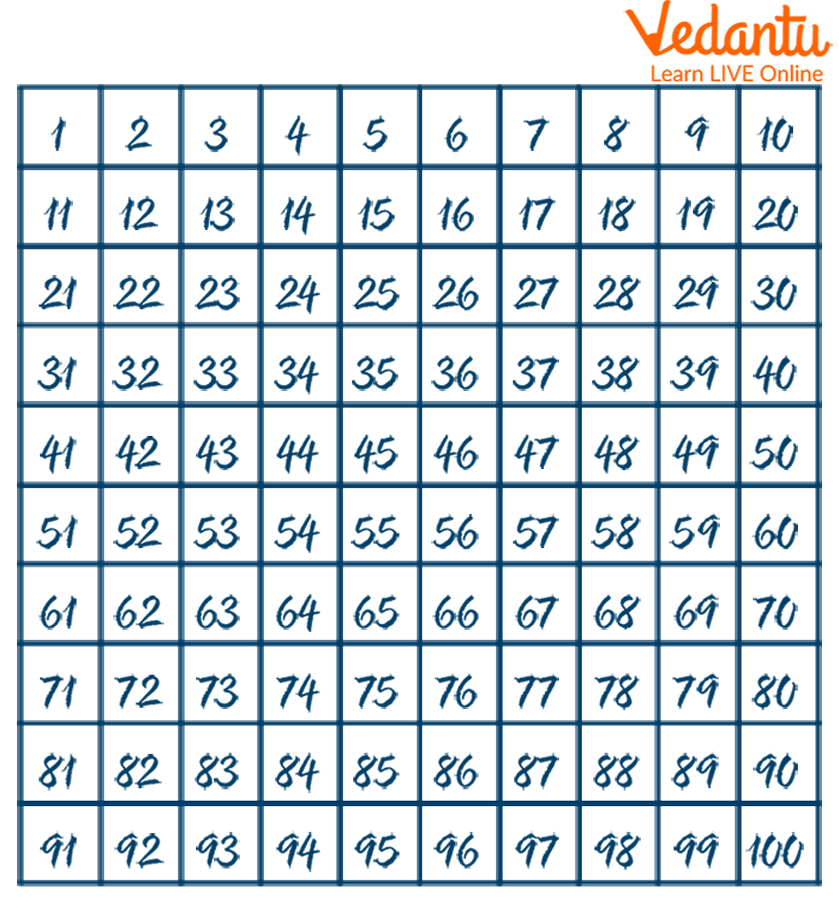Counting Numbers (by 1)

## Properties of Skip Counting by 3

Suppose we dive into counting from zero (0) itself, following skip counting by 3, the first number we would land on is 3, then we will skip 4 and 5, and the next number is 6. The counting, by 1, 2, 3, or others, all can be done in two patterns, forward and backward both. Suppose we have a chart of numbers from 1 to 30 and we do forward skip count by 3 from 0, then the numbers will be 3, 6, 9, 12, and so on. Now, with the same chart of numbers, we do a backward skip count by 3 from 30, then the numbers will be 27, 24, 21, 18, and so on.

## Skip Counting by 3 Chart

Among the many ways in which skip counting by 3 can be visualised, representing through a chart is also an option. In the chart, every third number will be highlighted to make it easy to count by 3. Here is the skip counting by 3 chart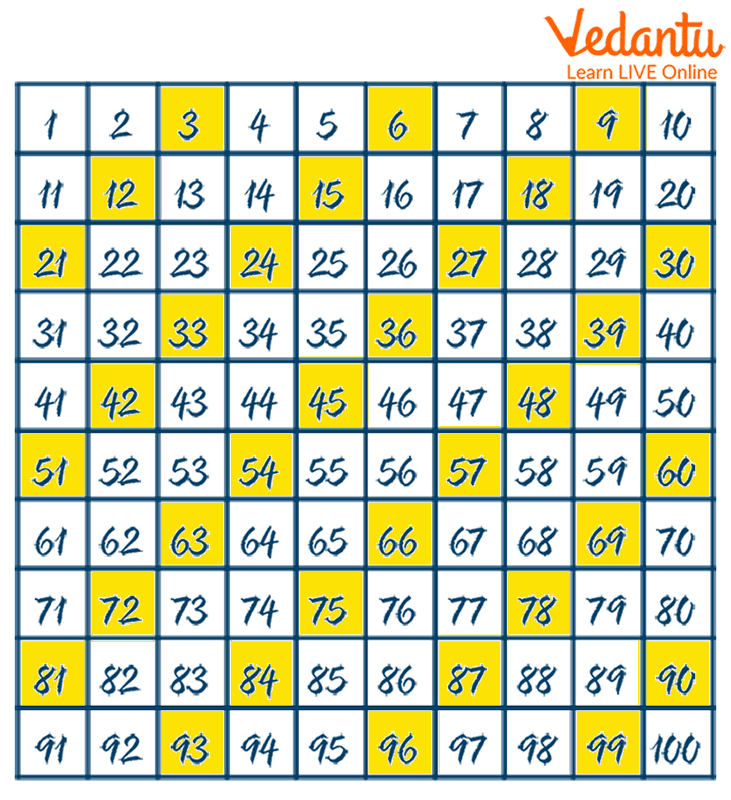Skip Counting by 3 Chart

From the chart, it can be seen that by highlighting every third number, a diagonal pattern has been formed and this diagonal pattern also represents the multiplication table of 3.

## How to Count by 3 on the Number Line?

How to count by 3 is a question that can also be solved through the number line. We consider a basic number line starting from 0. Now, we know that to skip count by 3, we leave two numbers and jump to the third number directly. Starting from 0, we will skip 1, 2, and jump to 3, then we will skip 4, 5, and jump to 6. So, the number line will look like this: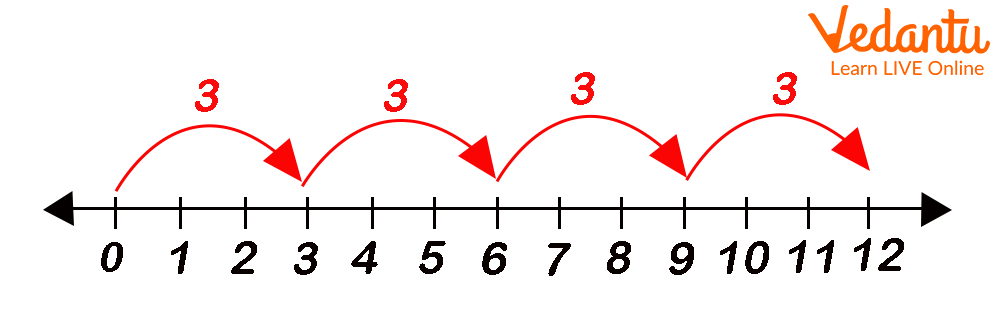Skip Counting by 3 on the Number Line

## Patterns Formed by Skip Counting Numbers

When skipping counting numbers, there is always a pattern followed. We will study it through some examples:

• When we skip count by 2, the resultant series will be 2, 4, 6, 8, 10, and so on. If we show this series on the chart, a skip counting pattern will be formed.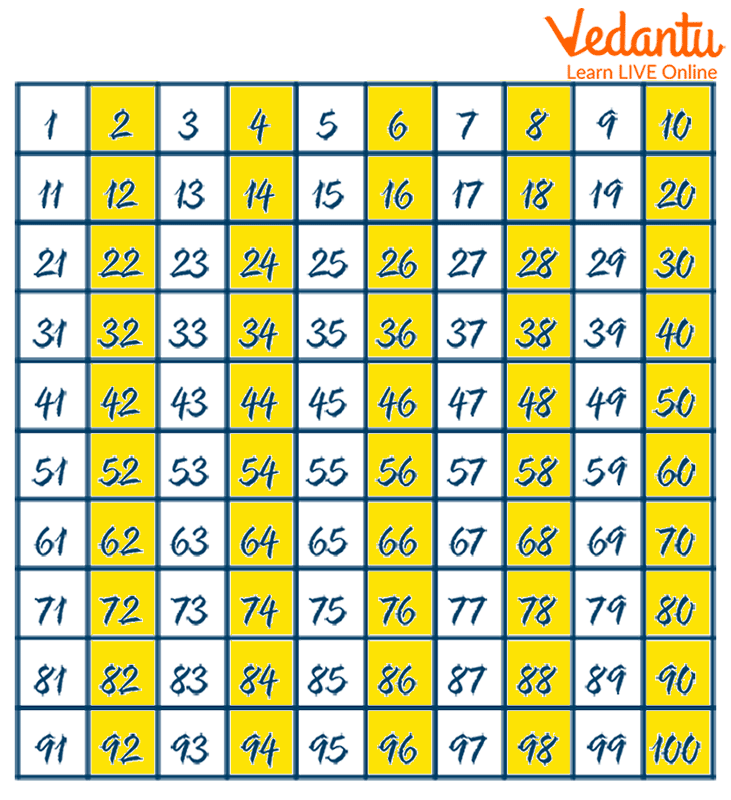Skip Counting by 2

We can see that here, a vertical line pattern is formed.

• When we skip count by 9, the resultant series will be 9, 18, 27, 36, and so on. If we show this series on the chart, a skip counting pattern will be formed.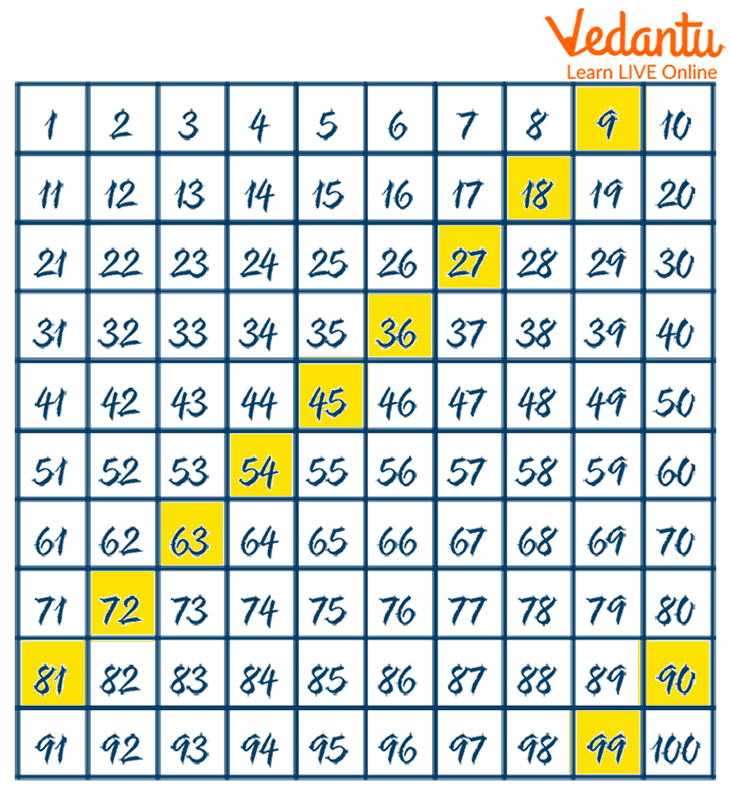Skip Counting by 9

We can see that here, a diagonal pattern is formed.

Similarly, skip counting patterns are formed in many series and it makes the addition and multiplication tables easy to learn for kids.

## Solved Examples

1. Complete the skip counting series.

96, 93, __, 87, __

Considering the series, we can see that backward counting has been done.

\[96 - 3 = 93\]

So,\[93 - 3 = 90\]

\[90 - 3 = 87\]

So, \[87 - 3 = 84\]

The complete series is \[96, 93, 90, 87, 84.\]

2. Find the skip counting 3 starting from 30 series.

Starting from 30, the skip count by 3:

\[30 + 3 = 33\]

\[33 + 3 = 36\]

\[36 + 3 = 39\]

\[39 + 3 = 42\]

So, the required series will be 30, 33, 36, 39, 42, ......

## Conclusion

Skip counting 3 is done by skipping two numbers in between and jumping into the third number directly. It displays a multiplication table of 3 and is basic learning for addition. It can be done both forward and backward."Counting is the process of expressing the number of components or objects that are supplied," according to the definition of counting. While skipping counting, we omit some of the numbers as we count. When counting backwards by threes, we omit the first two digits and jump straight to the third. To skip count by three, add three to each number to get the following one in the sequence. This technique aids in understanding additional concepts and memorization of the three-step table.

Last updated date: 19th Sep 2023
Total views: 80.7k
Views today: 2.80k

## FAQs on Skip Counting By 3

1. How to count by 3?

To count by 3, we skip the first two numbers from the starting point and jump to the third number directly.

2. What pattern does skip counting by 3 forms?

Visualising skip counting by 3 on the chart, skip count by 3 forms a diagonal pattern.

3. Can skip counting by 3 be done forward and backward both?

Yes, we can skip count by 3, both forward and backward.

4. What is skip counting?

It refers to skipping the numbers in between and jumping onto the desired digit. For example, in skip counting by 3, we skip 2 digits and jump to the third.

5. What do students learn by skipping counting?

Skip counting increases the ability to see patterns in the number series given.